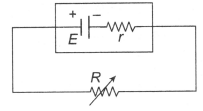In an electric circuit shown in the figure, the external resistance R is variable. It is found that same power is developed in external resistance R if its value is either 5  Ω  or  20  Ω.  With this information find the internal resistance (r) of the voltage source.

# In an electric circuit shown in the figure, the external resistance R is variable. It is found that same power is developed in external resistance R if its value is either $5\text{\hspace{0.17em}\hspace{0.17em}}\mathrm{\Omega }\text{\hspace{0.17em}\hspace{0.17em}}\mathrm{or}\text{\hspace{0.17em}\hspace{0.17em}}20\text{\hspace{0.17em}\hspace{0.17em}}\mathrm{\Omega }$.  With this information find the internal resistance (r) of the voltage source.1. A

$20\text{\hspace{0.17em}\hspace{0.17em}}\Omega$

2. B

$8\text{\hspace{0.17em}\hspace{0.17em}}\Omega$

3. C

$103\text{\hspace{0.17em}\hspace{0.17em}}\Omega$

4. D

$10\text{\hspace{0.17em}\hspace{0.17em}}\Omega$

Register to Get Free Mock Test and Study Material

+91

Verify OTP Code (required)

### Solution:

$\begin{array}{l}\mathrm{I}=\text{\hspace{0.17em}\hspace{0.17em}\hspace{0.17em}}\frac{\mathrm{E}}{\mathrm{R}+\mathrm{r}},\text{\hspace{0.17em}\hspace{0.17em}}\mathrm{P}={\mathrm{I}}^{2}\mathrm{R}=\frac{{\mathrm{E}}^{2}\mathrm{R}}{{\left(\mathrm{R}+\mathrm{r}\right)}^{2}}\\ \mathrm{Now}\text{\hspace{0.17em}\hspace{0.17em}}{\mathrm{P}}_{1}={\mathrm{P}}_{2}\text{\hspace{0.17em}}⇒\text{\hspace{0.17em}\hspace{0.17em}}\frac{{\mathrm{E}}^{2}5}{{\left(5+\mathrm{r}\right)}^{2}}\text{\hspace{0.17em}}=\text{\hspace{0.17em}\hspace{0.17em}}\frac{{\mathrm{E}}^{2}20}{{\left(20+\mathrm{r}\right)}^{2}}\text{\hspace{0.17em}}⇒\text{\hspace{0.17em}}\mathrm{r}=10\text{\hspace{0.17em}}\mathrm{\Omega }\end{array}$Register to Get Free Mock Test and Study Material

+91

Verify OTP Code (required)